# Dividing Decimals

Dividing decimals is similar to the division of whole numbers except for the decimals point. A similar division approach (like we do for whole numbers) is followed for decimals in maths. Before understanding the division of decimals, let’s recall what decimals and the meaning of decimal points are. In Mathematics, decimals are specific types of numbers, with a whole number and the fractional part separated by a decimal point. The dot that exists between the whole number and fractions part is called the decimal point. We can also perform various arithmetic operations on these numbers, such as addition, subtraction, multiplication and division.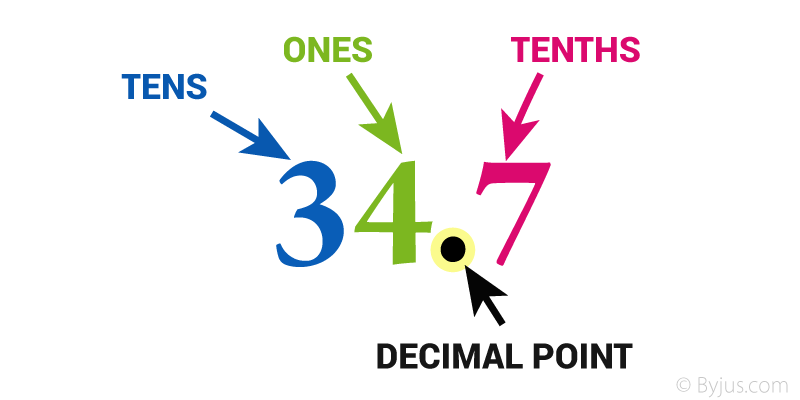## What is the Division of Decimals?

Dividing Decimals is similar to dividing whole numbers, except for the way we handle the decimal point. There are different cases in dividing decimals, such as:

• Dividing Decimals by whole numbers
• Division of a Decimal Number by another Decimal Number
• Division of decimals by 10, 100 and 1000

However, we may get a decimal or whole number when we divide decimal numbers. Let’s understand the division of these decimals in detail here.

## Dividing Decimals by whole numbers

There will be certain rules for dividing decimals in maths. These can be observed from the illustrative examples below.

The step wise procedure for dividing a decimal number by a whole number is given below for 1926 ÷ 4:

Step 1: Write the given division expression in the standard form.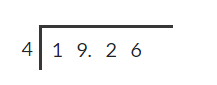Step 2: Now, we have to divide the decimal number’s whole number part by the divisor.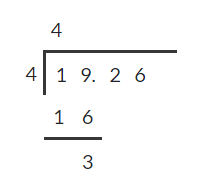Step 3: Place the decimal point in the quotient above the dividend’s decimal point and then bring down the tenth digit.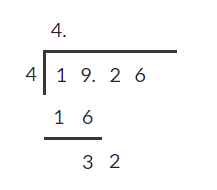Step 4: If the tenths digit of the obtained dividend cannot be divided by the divisor, write 0 in the quotient and in front of the tenths digit. Otherwise, proceed with the division process.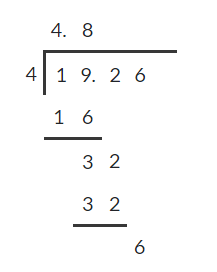Step 5: Repeat the above step and keep adding the zeroes in the dividend until 0 is obtained in the remainder.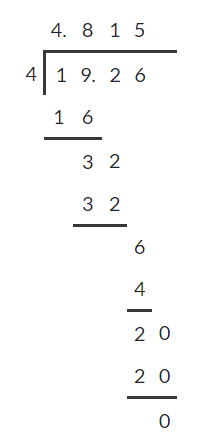Therefore, 19.26 ÷ 4 = 4.815

That means, the quotient is 4.815 and the remainder is 0.

In this example, we considered only those divisions in which the number would be completely divisible by another number to give the remainder zero. However, there are some situations where the given decimal number may not be completely divisible by another number, i.e., we may not get a remainder zero. This can be understood from the example given below.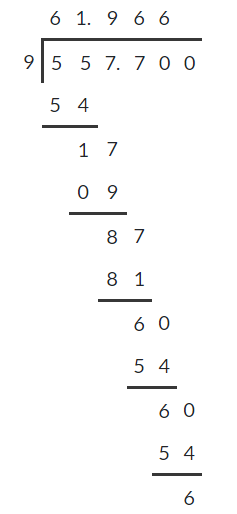## Division of a Decimal Number by another Decimal Number

When we divide decimals, we have to convert the divisor to a whole number by moving the decimal point to the right. Then, we carry the dividend’s decimal point up to the same number of places to the right and divide the resultant numbers in the usual way as we perform in regular long division. This can be better understood with the help of the following example.

23.184 ÷ 0.3

The given Divisor = 0.3 and Dividend = 23.184

Change the divisor 0.3 to a whole number by moving the decimal point 1 place to the right. Then move the decimal point in the dividend the same, 1 place to the right.

23.184 ÷ 0.3 = 231.84 ÷ 3

Now, divide the decimal number 231.84 by the whole number., i.e. 3.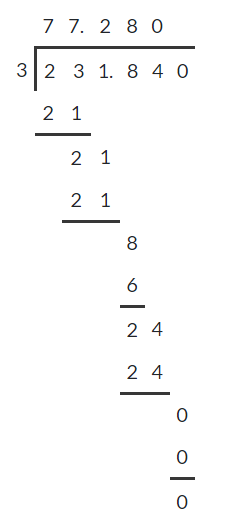### Dividing decimals by 10, 100 and 1000

Let us find the division of a decimal number by 10, 100 and 1000.

Consider 97.5 ÷ 10 = 9.75. Thus, the digits in 97.5 and 9.75 are the same, i.e., 9, 7, and 5, but the decimal point has shifted (towards left) in the quotient. Therefore, in a decimal division by 10, the decimal point will shift to the left by one place since 10 has only one zero over 1.

From the above example, we can say that in the division of decimals by 10, 100 or 1000, the number and quotient digits will be the same. However, the decimal point in the quotient shifts to the left by as many places as zeros over 1.

### Dividing Decimals examples

The below solved examples help you in better understanding of the division of decimal numbers.

Example 1: Find the quotient for 1.764 ÷ 0.012.

Solution:

The given Divisor = 0.012 and Dividend = 1.764

Now, change the divisor 0.012 to a whole number by moving the decimal point 3 places to the right. Then move the decimal point in the dividend the same, 3 places to the right.

1.764 ÷ 0.012 = 1764.0 ÷ 12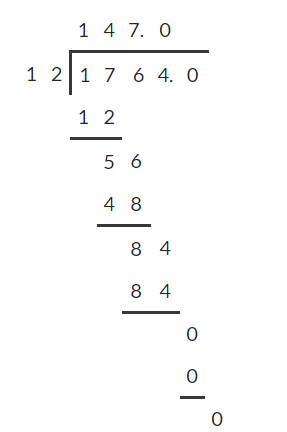Thus, the quotient is 147.

Example 2: Calculate: 8.91 ÷ 2.2

Solution:

The given Divisor = 2.2 and Dividend = 8.91

Now, change the divisor 2.2 to a whole number by moving the decimal point 1 place to the right. Then move the decimal point in the dividend the same, 1 place to the right.

8.91 ÷ 2.2 = 8910 ÷ 22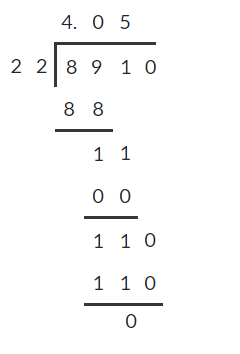### Dividing Decimals Problems

Solve the below practice problems on decimal division.

1. Divide 0.4545 by 3.6.

2. Find the remainder when 651.2 is divided by 0.04.

3. Evaluate: 7.75 ÷ 0.0025

4. Find the quotient of 82.44 ÷ 18.

## Frequently Asked Questions – FAQs

### How do you divide decimals step by step?

The step-wise procedure for dividing decimals by a whole number is given below:
Step 1: Write the given division expression in the standard form.
Step 2: Now, we have to divide the decimal number’s whole number part by the divisor.
Step 3: Place the decimal point in the quotient above the dividend’s decimal point and then bring down the tenths’ digit.
Step 4: If the tenth digit of the obtained dividend cannot be divided by the divisor, write 0 in the quotient and in front of the tenths’ digit. Otherwise, proceed with the division process.
Step 5: Repeat the above step and keep adding the zeroes in the dividend until 0 is obtained in the remainder.

### Which way do you move the decimal point when dividing by 10?

Dividing a decimal by 10 moves the decimal point one place to the left.

### How do you solve 5 divided by 100?

Dividing 5 by 100 involves only placing the decimal point in the numerator, such as 5/100 = 0.05. That means the decimal point will shift to the left by two places since 100 has two 0’s.

### What is 31 over 100 as a decimal?

31 over 100 can be written as 31/100. Here, the denominator contains two 0’s. Hence, we have to place the decimal point after two digits, such as 0.31.

### How do you divide a decimal by 10?

When we divide a decimal number by 10, the decimal point will shift to the left by one place since 10 has only one zero over 1.